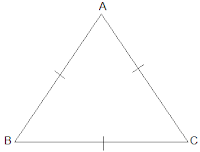Newbie

# Show that the angles of an equilateral triangle are 60° each. Q.8

• 0

Find the best solution of ncert class 9th chapter triangles . Please help me to solve this exercise 7.2 question number 8 . Find the best and easiest solution of this question , also find the easiest solution of this question.Show that the angles of an equilateral triangle are 60° each

Share

1. Let ABC be an equilateral triangle as shown below:Here, BC = AC = AB (Since the length of all sides is same)

⇒ A = B =C (Sides opposite to the equal angles are equal.)

Also, we know that

A+B+C = 180°

⇒ 3A = 180°

⇒ A = 60°

∴ A = B = C = 60°

So, the angles of an equilateral triangle are always 60° each.

• 0